# NCERT solutions for Class 7 Maths chapter 6 - The Triangle and its Properties [Latest edition]

#### Chapters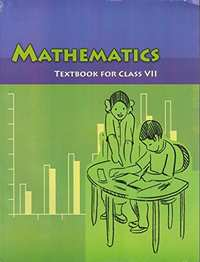## Chapter 6: The Triangle and its Properties

Exercise 6.1Exercise 6.2Exercise 6.3Exercise 6.4Exercise 6.5
Exercise 6.1 [Page 116]

### NCERT solutions for Class 7 Maths Chapter 6 The Triangle and its Properties Exercise 6.1 [Page 116]

Exercise 6.1 | Q 1 | Page 116

In ΔPQR, D is the mid-point of  bar(QR)

bar(PM) is _________

PD is __________

Is QM = MR?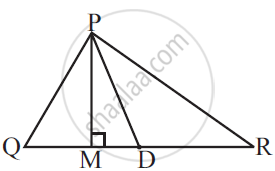Exercise 6.1 | Q 2.1 | Page 116

Draw rough sketches for the following

In ΔABC, BE is a median.

Exercise 6.1 | Q 2.2 | Page 116

Draw rough sketches for the following:

In ΔPQR, PQ and PR are altitudes of the triangle.

Exercise 6.1 | Q 2.3 | Page 116

Draw rough sketche for the following:

In ΔXYZ, YL is an altitude in the exterior of the triangle.

Exercise 6.1 | Q 3 | Page 116

Verify by drawing a diagram if the median and altitude of an isosceles triangle can be same.

Exercise 6.2 [Pages 118 - 119]

### NCERT solutions for Class 7 Maths Chapter 6 The Triangle and its Properties Exercise 6.2 [Pages 118 - 119]

Exercise 6.2 | Q 1.1 | Page 118

Find the value of the unknown exterior angle x in the Given diagrams: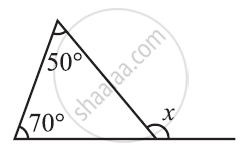Exercise 6.2 | Q 1.2 | Page 118

Find the value of the unknown exterior angle x in the Given diagrams: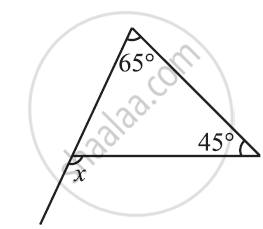Exercise 6.2 | Q 1.3 | Page 118

Find the value of the unknown exterior angle x in the Given diagrams: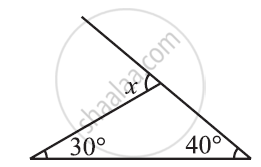Exercise 6.2 | Q 1.4 | Page 118

Find the value of the unknown exterior angle x in the Given diagrams: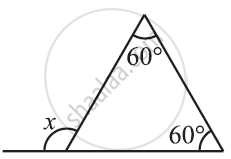Exercise 6.2 | Q 1.5 | Page 118

Find the value of the unknown exterior angle x in the Given diagrams: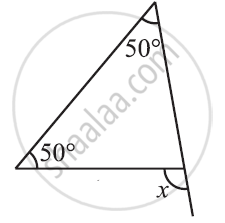Exercise 6.2 | Q 1.6 | Page 118

Find the value of the unknown exterior angle x in the Given diagrams: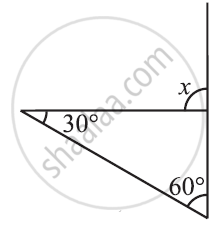Exercise 6.2 | Q 2.1 | Page 119

Find the value of the unknown interior angle x in the following figure.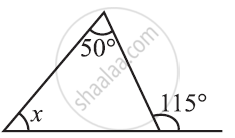Exercise 6.2 | Q 2.2 | Page 119

Find the value of the unknown interior angle x in the following figure.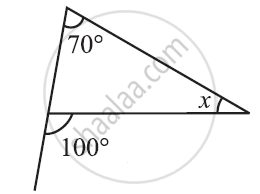Exercise 6.2 | Q 2.3 | Page 119

Find the value of the unknown interior angle x in the following figure.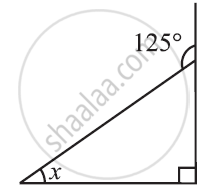Exercise 6.2 | Q 2.4 | Page 119

Find the value of the unknown interior angle x in the following figure.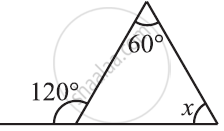Exercise 6.2 | Q 2.5 | Page 119

Find the value of the unknown interior angle x in the following figure.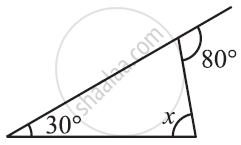Exercise 6.2 | Q 2.6 | Page 119

Find the value of the unknown interior angle x in the following figure.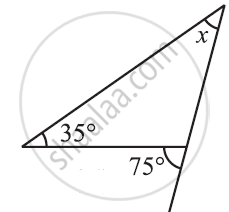Exercise 6.3 [Page 121]

### NCERT solutions for Class 7 Maths Chapter 6 The Triangle and its Properties Exercise 6.3 [Page 121]

Exercise 6.3 | Q 1.1 | Page 121

Find the value of the unknown x in the given diagram: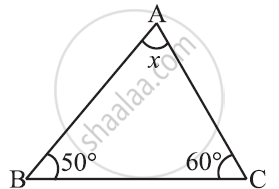Exercise 6.3 | Q 1.2 | Page 121

Find the value of the unknown x in the given diagram: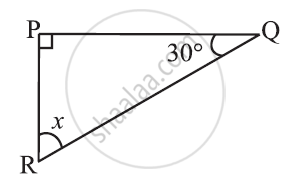Exercise 6.3 | Q 1.3 | Page 121

Find the value of the unknown x in the given diagram: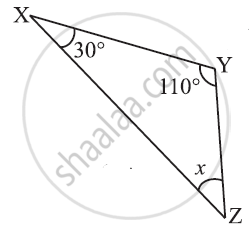Exercise 6.3 | Q 1.4 | Page 121

Find the value of the unknown x in the given diagram: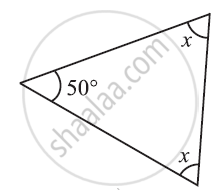Exercise 6.3 | Q 1.5 | Page 121

Find the value of the unknown x in the given diagram: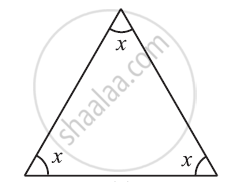Exercise 6.3 | Q 1.6 | Page 121

Find the value of the unknown x in the given diagram: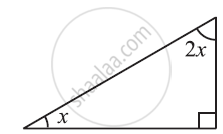Exercise 6.3 | Q 2.1 | Page 121

Find the value of the unknowns x and y in the following diagrams: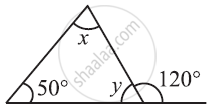Exercise 6.3 | Q 2.2 | Page 121

Find the value of the unknowns x and y in the following diagrams: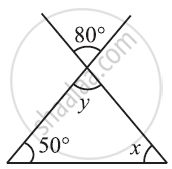Exercise 6.3 | Q 2.3 | Page 121

Find the value of the unknowns x and y in the following diagram: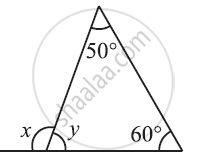Exercise 6.3 | Q 2.4 | Page 121

Find the value of the unknowns x and y in the following diagram: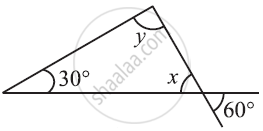Exercise 6.3 | Q 2.5 | Page 121

Find the value of the unknowns x and y in the following diagram: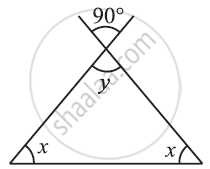Exercise 6.3 | Q 2.6 | Page 121

Find the value of the unknowns x and y in the following diagram: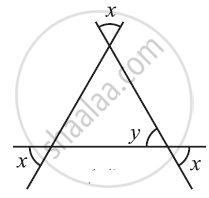Exercise 6.4 [Pages 126 - 127]

### NCERT solutions for Class 7 Maths Chapter 6 The Triangle and its Properties Exercise 6.4 [Pages 126 - 127]

Exercise 6.4 | Q 1.1 | Page 126

Is it possible to have a triangle with the following sides?

2 cm, 3 cm, 5 cm

Exercise 6.4 | Q 1.2 | Page 126

Is it possible to have a triangle with the following sides?

3 cm, 6 cm, 7 cm

Exercise 6.4 | Q 1.3 | Page 126

Is it possible to have a triangle with the following sides?

6 cm, 3 cm, 2 cm

Exercise 6.4 | Q 2 | Page 126

Take any point O in the interior of a triangle PQR. Is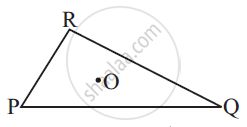1) OP + OQ > PQ?

2) OQ + OR > QR?

3) OR + OP > RP?

Exercise 6.4 | Q 3 | Page 126

AM is a median of a triangle ABC.

Is AB + BC + CA > 2 AM?

(Consider the sides of triangles ΔABM and ΔAMC.)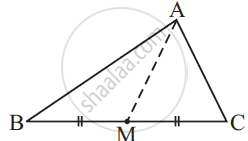Exercise 6.4 | Q 4 | Page 126

Is AB + BC + CD + DA >AC + BD?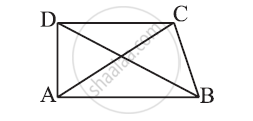Exercise 6.4 | Q 5 | Page 126

Is AB + BC + CD + DA < 2 (AC + BD)?

Exercise 6.4 | Q 6 | Page 127

The lengths of two sides of a triangle are 12 cm and 15 cm. Between what two measures should the length of the third side fall?

Exercise 6.5 [Page 130]

### NCERT solutions for Class 7 Maths Chapter 6 The Triangle and its Properties Exercise 6.5 [Page 130]

Exercise 6.5 | Q 1 | Page 130

PQR is a triangle right angled at P. If PQ = 10 cm and PR = 24 cm, find QR.

Exercise 6.5 | Q 2 | Page 130

ABC is a triangle right angled at C. If AB = 25 cm and AC = 7 cm, find BC.

Exercise 6.5 | Q 3 | Page 130

A 15 m long ladder reached a window 12 m high from the ground on placing it against a wall at a distance a. Find the distance of the foot of the ladder from the wall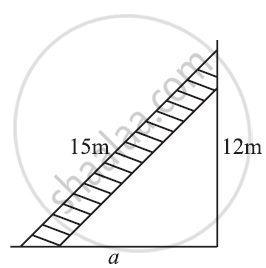Exercise 6.5 | Q 4.1 | Page 130

Which of the following can be the sides of a right triangle?

2.5 cm, 6.5 cm, 6 cm

In the case of right-angled triangles, identify the right angles.

Exercise 6.5 | Q 4.2 | Page 130

Which of the following can be the sides of a right triangle?

2 cm, 2 cm, 5 cm

In the case of right-angled triangles, identify the right angles.

Exercise 6.5 | Q 4.3 | Page 130

Which of the following can be the sides of a right triangle?

1.5 cm, 2 cm, 2.5 cm

In the case of right-angled triangles, identify the right angles.

Exercise 6.5 | Q 5 | Page 130

A tree is broken at a height of 5 m from the ground and its top touches the ground at a distance of 12 m from the base of the tree. Find the original height of the tree.

Exercise 6.5 | Q 6 | Page 130

Angles Q and R of a ΔPQR are 25° and 65°.

Write which of the following is true:

(1) PQ2 + QR2= RP2

(2) PQ2 + RP2= QR2

(3) RP2 + QR2= PQ2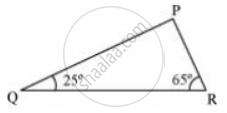Exercise 6.5 | Q 7 | Page 130

Find the perimeter of the rectangle whose length is 40 cm and a diagonal is 41 cm

Exercise 6.5 | Q 8 | Page 130

The diagonals of a rhombus measure 16 cm and 30 cm. Find its perimeter.

## Chapter 6: The Triangle and its Properties

Exercise 6.1Exercise 6.2Exercise 6.3Exercise 6.4Exercise 6.5## NCERT solutions for Class 7 Maths chapter 6 - The Triangle and its Properties

NCERT solutions for Class 7 Maths chapter 6 (The Triangle and its Properties) include all questions with solution and detail explanation. This will clear students doubts about any question and improve application skills while preparing for board exams. The detailed, step-by-step solutions will help you understand the concepts better and clear your confusions, if any. Shaalaa.com has the CBSE Class 7 Maths solutions in a manner that help students grasp basic concepts better and faster.

Further, we at Shaalaa.com provide such solutions so that students can prepare for written exams. NCERT textbook solutions can be a core help for self-study and acts as a perfect self-help guidance for students.

Concepts covered in Class 7 Maths chapter 6 The Triangle and its Properties are Concept of Triangles - Sides, Angles, Vertices, Interior and Exterior of Triangle, Isosceles Triangles, Median of a Triangle, Classification of Triangles (On the Basis of Sides, and of Angles), Altitudes of a Triangle, Exterior Angle of a Triangle and Its Property, Angle Sum Property of a Triangle, Sum of the Lengths of Two Sides of a Triangle, Right-angled Triangles and Pythagoras Property, Equilateral Triangle, Scalene Triangle, Acute Angled Triangle, Obtuse Angled Triangle, Right Angled Triangle, Some Special Types of Triangles - Equilateral and Isosceles Triangles.

Using NCERT Class 7 solutions The Triangle and its Properties exercise by students are an easy way to prepare for the exams, as they involve solutions arranged chapter-wise also page wise. The questions involved in NCERT Solutions are important questions that can be asked in the final exam. Maximum students of CBSE Class 7 prefer NCERT Textbook Solutions to score more in exam.

Get the free view of chapter 6 The Triangle and its Properties Class 7 extra questions for Class 7 Maths and can use Shaalaa.com to keep it handy for your exam preparation